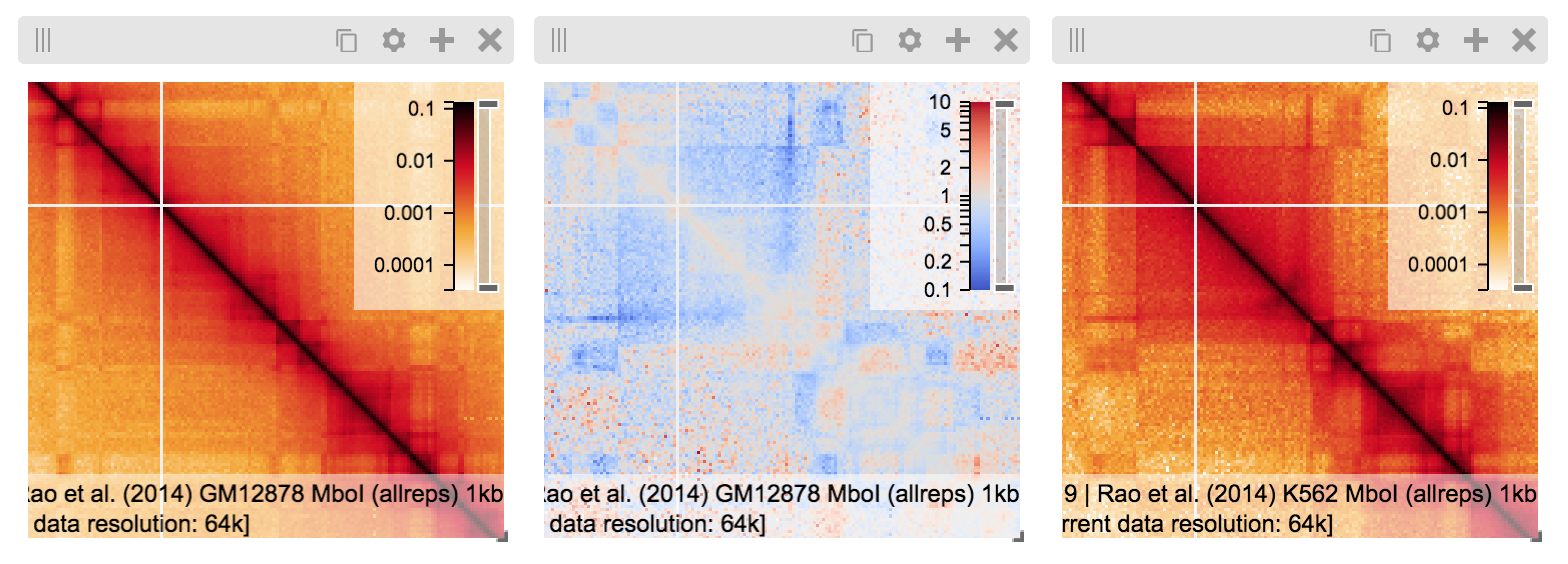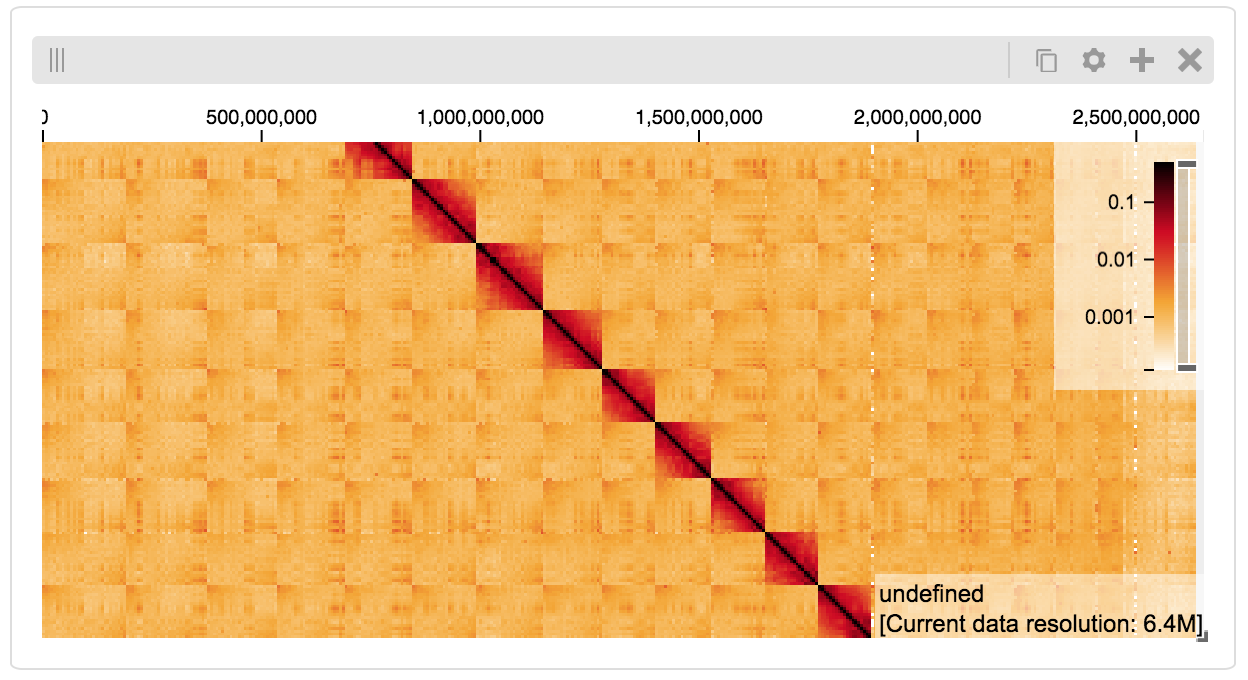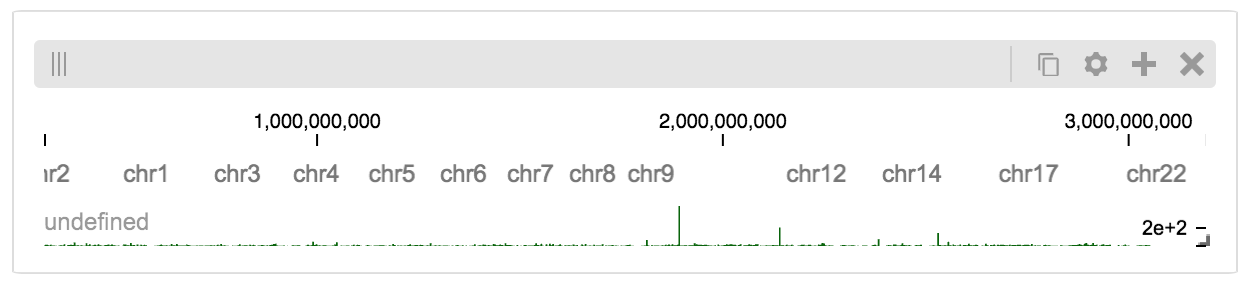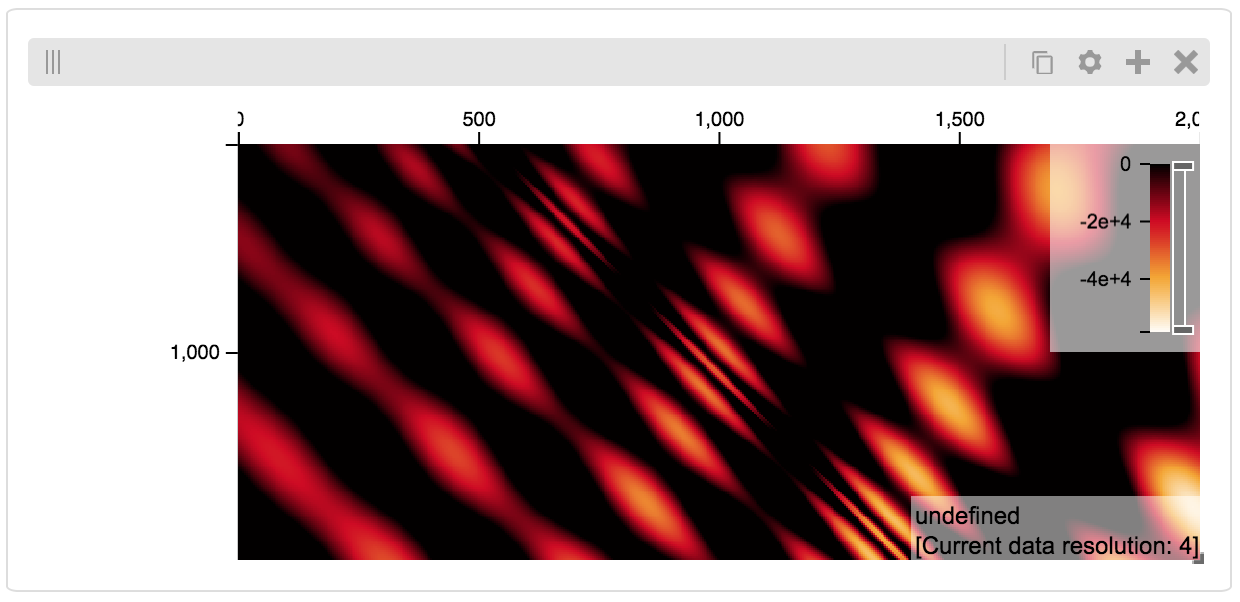# Getting Started¶

Python Jupyter notebooks are an excellent way to experiment with data science and visualization. Using the higlass-jupyter extension, you can use HiGlass directly from within a Jupyter notebook.

## Installation¶

To use higlass within a Jupyter notebook you need to install a few packages and enable the jupyter extension:

```pip install jupyter higlass-python

jupyter nbextension install --py --sys-prefix --symlink higlass
jupyter nbextension enable --py --sys-prefix higlass
```

If you use JupyterLab you also have to run

```jupyter labextension install @jupyter-widgets/jupyterlab-manager
jupyter labextension install higlass-jupyter
```

### Uninstalling¶

```jupyter nbextension uninstall --py --sys-prefix higlass
```

## Simplest Use Case¶

The simplest way to instantiate a HiGlass instance to create a display object with one view:

```import higlass
from higlass.client import Track, View

display, server, viewconf = higlass.display([View([Track('top-axis')])])
display
```

If brevity is of importance, the constructor for `View` can be omitted and a view will automatically be created from the list of Tracks: `higlass.display([[Track('top-axis')]])`. This, however, precludes the use of parameters with the view or for linking views using syncs. It also always uses the default position for a given track type.

## Remote Jupyter Notebook¶

If your Jupyter notebook is running on a remote server (at e.g. `REMOTE_IP`), you’ll need to make sure that you have an extra port (let’s call it `HG_PORT`) open on your firewall that HiGlass can use to communicate with its server. That port will then need to be passed to the server display command:

```higlass.display(
...,
server_port=HG_PORT,
host=REMOTE_IP,
fuse=False
)
```

The `fuse=False` option is often necessary if there is no support for FUSE. FUSE is only necessary for loading remote http datasets which are not hosted on a HiGlass server.

## View extent¶

The extent of a view can be set using the `initialXDomain` parameter:

```view1 = View([
Track(type='top-axis'),
], initialXDomain=[0,1e7])
```

## Track Types¶

A list of available track types can be found in the documentation for HiGlass. Based on the data type, we can sometimes provide a recommended track type as well as a recommended position.

```import higlass.client as hgc
track_type, position = hgc.datatype_to_tracktype(datatype)
```

## Color Maps¶

Certain quantative tracks such as the heatmap can vary their colormap. Color maps can be passed in directly as arrays of color values:

```Track('heatmap', tileset, colorRange=['white', 'black'])
```

Or created from a matplotlib colormap (`reversed=True` reverses the color order in the heatmap):

```from higlass.utils import hg_cmap
Track('heatmap', tileset, colorRange=hg_cmap('jet', reverse=True))
```

A list of available matplotlib color maps can be found in the matplotlib docs.

## Combining Tracks¶

Tracks can be combined by overlaying them on top of each other or by performing operations with them.

### Overlaying tracks¶

Two tracks can be overlayed by using the `+` operator:

```view=View([Track('top-axis') +
Track('horizontal-bar',
server='//higlass.io/api/v1',
tilesetUid='F2vbUeqhS86XkxuO1j2rPA')
], initialXDomain=[0,1e9])
```

Another way to express this is to pass in a list of tracks as if it were a single track:

```view=View([[Track('top-axis'),
Track('horizontal-bar',
server='//higlass.io/api/v1',
tilesetUid='F2vbUeqhS86XkxuO1j2rPA')
]], initialXDomain=[0,1e9])
```

## Multiple Views¶

Multiple views can be instantiated much like single views. They are positioned a on grid that is 12 units wide and an arbitrary number of units high. To create two side by side views, set both to be 6 units wide and one on the right to be at x position 6:

```import higlass
from higlass.client import Track, View

view1 = View([Track(type='top-axis')], x=0, width=6)
view2 = View([Track(type='top-axis')], x=6, width=6)

display, server, viewconf = higlass.display([view1, view2])
display
```## Synchronization¶

Views and track can be synchronized by location, zoom level and values scales.

### Zoom and Location locks¶

Location locks ensure that when one view is panned, all synchronized views pan with it. Zoom locks do the same with zoom level. Both can be instantiated by passing lists of views to lock to `higlass.display`. Each set of locked views will scroll or zoom (or both) together:

```display, server, viewconf = higlass.display(
[view1, view2],
location_syncs=[[view1, view2]],
zoom_syncs=[[view1, view2]])
```

## Viewport Projection¶

Viewport projections can be instantiated like other tracks. It is created with a reference to the view we wish to track and combined with another track where it will be overlayed.

```from higlass.client import ViewportProjection

view1 = View([
Track(type='top-axis'),
], initialXDomain=[0,1e7])

projection = ViewportProjection(view1)

view2 = View([
Track(type='top-axis') + projection,
], initialXDomain=[0,2e7])
```

Note that ViewportProjection tracks always need to be paired with other non- ViewportProjection tracks. Multiple ViewportProjection tracks can, however, be combined, as long as they are associated with regular tracks.

Combined tracks can also be created by passing a list of tracks as if it were a track itself to a `View`.

```view2 = View([
[ Track(type='top-axis'), projection ]
], initialXDomain=[0,2e7])
```

## Dataset Arithmetic¶

HiGlass supports client-side division between quantitative datasets. This makes it possible to quickly compare two datasets by visualizing their ratio as computed on loaded tiles rather than the entire dataset:

```t1 = Track(**track_def)
t2 = Track(**{ **track_def, "tileset_uuid": "QvdMEvccQuOxKTEjrVL3wA" })
t3 = t1 / t2
```

They can also be created using a constructor:

```from higlass.client import DividedTrack

t3 = DividedTrack(t1, t2)
```

The full example is here:

```from higlass.utils import hg_cmap

track_def = {
"track_type": 'heatmap',
"position": 'center',
"tileset_uuid": 'CQMd6V_cRw6iCI_-Unl3PQ',
"server": "http://higlass.io/api/v1/",
"height": 210,
"options": {}
}

t1 = Track(**track_def)
t2 = Track(**{ **track_def, "tileset_uuid": "QvdMEvccQuOxKTEjrVL3wA" })
t3 = (t1 / t2).change_attributes(
options={
'colorRange': hg_cmap('coolwarm'),
'valueScaleMin': 0.1,
'valueScaleMax': 10,
})
domain = [7e7,8e7]

v1 = View([t1], x=0, width=4, initialXDomain=domain)
v2 = View([t3], x=4, width=4, initialXDomain=domain)
v3 = View([t2], x=8, width=4, initialXDomain=domain)

display, server, viewconf = higlass.display([v1, v2, v3])
display
```## Saving the view¶

```display.save_as_png('/tmp/my_view.png')
```

Not that this function can only be used within a Jupyter notebook and works asynchronously so the saved screenshot will not nessarily be complete immediately after the function finishes executing

## Authorization¶

If loading tiles from a secured server, the `auth_token` parameter takes the string that will be used as the Authorization header on all tile requests sent out by HiGlass:

```(d,s,v) = higlass.display(views, auth_token='JWT DEADBEEF')
```

## Other Examples¶

The examples below demonstrate how to use the HiGlass Python API to view data locally in a Jupyter notebook or a browser-based HiGlass instance.

For a more complete overview, you can find the demos from the talk at github.com/higlass/scipy19.

### Jupyter HiGlass Component¶

To instantiate a HiGlass component within a Jupyter notebook, we first need to specify which data should be loaded. This can be accomplished with the help of the `higlass.client` module:

```from higlass.client import View, Track
import higlass

view1 = View([
Track(track_type='top-axis', position='top'),
Track(track_type='heatmap', position='center',
tileset_uuid='CQMd6V_cRw6iCI_-Unl3PQ',
server="http://higlass.io/api/v1/",
height=250,
options={ 'valueScaleMax': 0.5 }),
])
```

### Remote bigWig Files¶

bigWig files can be loaded either from the local disk or from remote http servers. The example below demonstrates how to load a remote bigWig file from the UCSC genome browser’s archives. Note that this is a network-heavy operation that may take a long time to complete with a slow internet connection.

```from higlass.client import View, Track
import higlass.tilesets

ts1 = higlass.tilesets.bigwig(
'wgEncodeSydhTfbs/wgEncodeSydhTfbsGm12878InputStdSig.bigWig')

tr1 = Track('horizontal-bar', tileset=ts1)
view1 = View([tr1])
display, server, viewconf = higlass.display([view1])

display
```

### Serving local data¶

To view local data, we need to define the tilesets and set up a temporary server.

#### Cooler Files¶

Creating the server:

```from higlass.client import View, Track
from higlass.tilesets import cooler
import higlass

ts1 = cooler('../data/Dixon2012-J1-NcoI-R1-filtered.100kb.multires.cool')
tr1 = Track('heatmap', tileset=ts1)
view1 = View([tr1])
display, server, viewconf = higlass.display([view1])

display
```#### BigWig Files¶

In this example, we’ll set up a server containing both a chromosome labels track and a bigwig track. Furthermore, the bigwig track will be ordered according to the chromosome info in the specified file.

```from higlass.client import View, Track
from higlass.tilesets import bigwig, chromsizes
import higlass.tilesets

chromsizes_fp = '../data/chromSizes_hg19_reordered.tsv'
bigwig_fp = '../data/wgEncodeCaltechRnaSeqHuvecR1x75dTh1014IlnaPlusSignalRep2.bigWig'

with open(chromsizes_fp) as f:
chromsizes = []
chrom, size = line.split('\t')
chromsizes.append((chrom, int(size)))

cs = chromsizes(chromsizes)
ts = bigwig(bigwig_fp, chromsizes=chromsizes)

tr0 = Track('top-axis')
tr1 = Track('horizontal-bar', tileset=ts)
tr2 = Track('horizontal-chromosome-labels', position='top', tileset=cs)

view1 = View([tr0, tr1, tr2])
display, server, viewconf = higlass.display([view1])

display
```

The client view will be composed such that three tracks are visible. Two of them are served from the local server.### Serving custom data¶

To display data, we need to define a tileset. Tilesets define two functions: `tileset_info`:

```> from higlass.tilesets import bigwig
> ts1.tileset_info()
{
'min_pos': ,
'max_pos': ,
'max_width': 4294967296,
'tile_size': 1024,
'max_zoom': 22,
'chromsizes': [['chr1', 249250621],
['chr2', 243199373],
...],
'aggregation_modes': {'mean': {'name': 'Mean', 'value': 'mean'},
'min': {'name': 'Min', 'value': 'min'},
'max': {'name': 'Max', 'value': 'max'},
'std': {'name': 'Standard Deviation', 'value': 'std'}},
'range_modes': {'minMax': {'name': 'Min-Max', 'value': 'minMax'},
'whisker': {'name': 'Whisker', 'value': 'whisker'}}
}
```

and `tiles`:

```> ts1.tiles(['x.0.0'])
[('x.0.0',
{'min_value': 0.0,
'max_value': 9.119079544037932,
'dense': 'Rh25PwcCcz...',   # base64 string encoding the array of data
'size': 1,
'dtype': 'float32'})]
```

The tiles function will always take an array of tile ids of the form `id.z.x[.y][.transform]` where `z` is the zoom level, `x` is the tile’s x position, `y` is the tile’s y position (for 2D tilesets) and `transform` is some transform to be applied to the data (e.g. normalization types like `ice`).

#### Numpy Matrix¶

By way of example, let’s explore a numpy matrix by implementing the tileset_info and tiles functions described above. To start let’s make the matrix using the Eggholder function.

```import numpy as np

dim = 2000
I, J = np.indices((dim, dim))
data = (
-(J + 47) * np.sin(np.sqrt(np.abs(I / 2 + (J + 47))))
- I * np.sin(np.sqrt(np.abs(I - (J + 47))))
)
```

Then we can define the data and tell the server how to render it.

```from  clodius.tiles import npmatrix
from higlass.tilesets import Tileset

ts = Tileset(
tileset_info=lambda: npmatrix.tileset_info(data),
tiles=lambda tids: npmatrix.tiles_wrapper(data, tids)
)

display, server, viewconf = higlass.display([
View([
Track(track_type='top-axis', position='top'),
Track(track_type='left-axis', position='left'),
Track(track_type='heatmap',
position='center',
tileset=ts,
height=250,
options={ 'valueScaleMax': 0.5 }),

])
])
display
```#### Displaying Many Points¶

To display, for example, a list of 1 million points in a HiGlass window inside of a Jupyter notebook. First we need to import the custom track type for displaying labelled points:

```%%javascript

require(["https://unpkg.com/higlass-labelled-points-track@0.1.11/dist/higlass-labelled-points-track"],
function(hglib) {

});
```

Then we have to set up a data server to output the data in “tiles”.

```import numpy as np
import pandas as pd
from higlass.client import View, Track
from higlass.tilesets import dfpoints

length = int(1e6)
df = pd.DataFrame({
'x': np.random.random((length,)),
'y': np.random.random((length,)),
'v': range(1, length+1),
})

ts = dfpoints(df, x_col='x', y_col='y')

display, server, viewconf = higlass.display([
View([
Track('left-axis'),
Track('top-axis'),
Track('labelled-points-track',
tileset=ts,
position='center',
height=600,
options={
'xField': 'x',
'yField': 'y',
'labelField': 'v'
}),
])
])

display
```This same technique can be used to display points in a GeoJSON file. First we have to extract the values from the GeoJSON file and create a dataframe:

```import math

def lat2y(a):
return 180.0/math.pi*math.log(math.tan(math.pi/4.0+a*(math.pi/180.0)/2.0))

x = [t['geometry']['coordinates'] for t in trees['features']]
y = [-lat2y(t['geometry']['coordinates']) for t in trees['features']]
names = [t['properties']['SPECIES'] for t in trees['features']]

df = pd.DataFrame({ 'x': x, 'y': y, 'names': names })
df = df.sample(frac=1).reset_index(drop=True)
```

And then create the tileset and track, as before.

```from higlass.client import View, Track
from higlass.tilesets import dfpoints

ts = dfpoints(df, x_col='x', y_col='y')

display, server, viewconf = higlass.display([
View([
Track('left-axis'),
Track('top-axis'),
Track('osm-tiles', position='center'),
Track('labelled-points-track',
tileset=ts,
position='center',
height=600,
options={
'xField': 'x',
'yField': 'y',
'labelField': 'names'
}),
])
])

display
```#### Other constructs¶

The examples containing dense data above use the bundled_tiles_wrapper_2d function to translate lists of tile_ids to tile data. This consolidates tiles that are within rectangular blocks and fulfills them simultaneously. The return type is a list of `(tile_id, formatted_tile_data)` tuples.

In cases where we don’t have such a function handy, there’s the simpler tiles_wrapper_2d which expects the target to fullfill just single tile requests:

```from clodius.tiles.format import format_dense_tile
from clodius.tiles.utils import tiles_wrapper_2d
from higlass.tilesets import Tileset

ts = Tileset(
tileset_info=tileset_info,
tiles=lambda tile_ids: tiles_wrapper_2d(tile_ids,
lambda z,x,y: format_dense_tile(tile_data(z, x, y)))
)
```

In this case, we expect tile_data to simply return a matrix of values.

## Troubleshooting¶

### Accessing the server log¶

A local server writes its log records to an in-memory StringIO buffer. The server’s name can be used to access its logger.

```import logging

logger = logging.getLogger(server.name)
logger.info('Hi!')

# convert the stream into a string
print(server.log.getvalue())

# write the log to a file
with open('higlass-server.log', 'wt') as f:
f.write(server.log.getvalue())
```